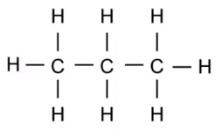Decane is an alkane with 10 carbons in a chain in it.  How many hydrogen atoms should it have?

Which of these is the standard “algebra” formula for an alkane?

What is the chemical formula for butane?

This is the structural formula of propane.  What is it’s regular formula?What is the simplest alkane molecule with the formula CH4 called?

What atoms are the only ones found in alkanes?